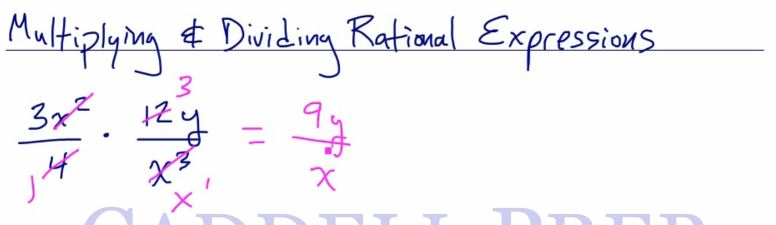In this video, we are going to multiply and divide rational expressions. After you finish this lesson, view all of our Algebra 1 lessons and practice problems.

We can start by either directly performing the operation or reduce across.

For example:$\frac{15}{4}\times\frac{32}{20}$

First, reduce the numbers that are across from each other$\dfrac{15}{4}\times\dfrac{32}{20}$$\dfrac{3}{1}\times\dfrac{8}{4}$

Further reduce, which can be done vertically with the 8 and the 4$\dfrac{3}{1}\times\dfrac{2}{1}$

Multiply$\dfrac{6}{1}$

And we have$6$

With variables, it is the same concept$\dfrac{3x+15}{x^2+7x+12}\times\dfrac{x^2-9}{x+5}$

Factor the expressions if necessary$\dfrac{3(x+5)}{(x+4)(x+3)}\times\dfrac{(x+3)(x+3)}{(x+5)}$

Reduce diagonally$\dfrac{3}{(x+4)}\times\dfrac{(x+3)}{1}$

Multiple across$\dfrac{3(x+3)}{x+4}$

Division is similar to multiplication.

Remember to Keep, Change, Flip

For example:$\dfrac{x+8}{3x}\div\dfrac{4x+32}{15x^4}$

Keep the first fraction, change the operation from division to multiplication, and flip the second fraction$\dfrac{x+8}{3x}\times\dfrac{15x^4}{4x+32}$

Factor if necessary$\dfrac{x+8}{3x}\times\dfrac{15x^4}{4(x+8)}$

Reduce expressions$\dfrac{1}{1}\times\dfrac{5x^3}{4}$

Multiply across$\dfrac{5x^3}{4}$

Examples of Multiplying And Dividing Rational Expressions

Example 1

Solve$\dfrac{5a^2}{14}\cdot\dfrac{7}{10a^3}$
First, multiply the numerators together, and multiply the denominators together$\dfrac{35a^2}{140a^3}$
Simplify by finding common factors in the numerator and denominator
So, cancel out$35$ and$a^2$$\dfrac{35 \cdot a^2}{4 \cdot 35 \cdot a^2\cdot a}$$\dfrac{1}{4a}$

Example 2$\dfrac{3x^2}{x+2}\div\dfrac{6x^4}{x^2+5x+6}$
First, lets factor out$x^2+5x+6$$\dfrac{3x^2}{x+2}\div\dfrac{6x^4}{(x+3)(x+2)}$
Now, in order to solve this, we do keep-change-flip.
Keep the first one. Then change to multiplication. And lastly, flip the second expression.$\dfrac{3x^2}{x+2}\times\dfrac{(x+3)(x+2)}{6x^4}$
Then, simplify by finding common factors in the numerator and denominator
So, cancel out$x+2$${3x^2}\times\dfrac{(x+3)}{6x^4}$
Now,$x^2$ is cancelled. Then$3$ and$6$$\dfrac{x+3}{3x^2}$

Video-Lesson Transcript

Let’s go over how to multiply and divide rational expressions.

For example:$\dfrac{3x^2}{4} \times \dfrac{12y}{x^3}$

Just like normal fractions, we can multiply straight across or cross-cancel first.

I advise you to do cross-cancel first.

Just a review,$\dfrac{15}{4} \times \dfrac{32}{20}$

We’ll cross-cancel by finding the greatest common factor of$4$ and$8$ – which is$4$.

Then identify what number goes into$15$ and$20$. So,$5$ does.$= \dfrac{3}{1} \times \dfrac{8}{4}$

Then, we can reduce the second expression and we’ll have$= \dfrac{3}{1} \times \dfrac{2}{1}$

Let’s multiply across$= \dfrac{6}{1}$$= 6$

Let’s do the same thing with our example.$\dfrac{3x^2}{4} \times \dfrac{12y}{x^3}$

Let’s see what we can reduce.$4$ and$12$ can be reduced.

Then let’s subtract the exponents of$x$.$= \dfrac{3}{1} \times \dfrac{3y}{x^1}$

Now, we can multiply across.$= \dfrac{9y}{x}$Let’s have a more complex example.$\dfrac{3x + 15}{x^2 + 7x + 12} \times \dfrac{x^2 - 9}{x + 5}$

Here, we can’t cross-cancel because the terms are being added and subtracted.

Because in cross-cancel, things are being multiplied.

An example of this is:$\dfrac{(3) (4)}{(5)} \times \dfrac{(10) (7)}{(6)}$

This can be cross-cancelled because everything is multiplied.

What if terms aren’t multiplied?

We have to factor them out first. So we can come up with multiplication.

Only then can we cross-cancel.$\dfrac{3 (x + 5)}{(x + 4) (x + 3)} \times \dfrac{(x + 3) (x - 3)}{(x + 5)}$

Now that we factored this, let’s see what we can reduce.

We can cancel$x + 5$ and$x +3$$\dfrac{3 (x - 3)}{x + 4}$

Now, let’s look at division.

Before we go into the lesson, let’s have a quick look at the division of fractions.$\dfrac{12}{5} \div \dfrac{2}{9}$

In order to solve this, we do keep-change-flip.

Keep the first one. Then change to multiplication. And lastly, flip the second expression.$\dfrac{12}{5} \times \dfrac{9}{2}$

Then follow the same rule in multiplication.

Cross-cancel if you can.$= \dfrac{6}{5} \times \dfrac{9}{1}$$= \dfrac{54}{5}$

Now, let’s apply the same rules to this problem.$\dfrac{x + 8}{3x} \div \dfrac{4x + 32}{15x^4}$

So let’s do keep-change-flip.

Keep the first one, change to multiplication and flip the last one.$= \dfrac{x + 8}{3x} \times \dfrac{15x^4}{4x + 32}$

Let’s try to factor this before we reduce.$= \dfrac{x + 8}{3x} \times \dfrac{15x^4}{4 (x + 8)}$

Now, let’s reduce.$x +8$ cancelled. Then$3$ and$15$. Then we’ll subtract the exponent of the$x$-es.

To get the final answer of$= \dfrac{5x^3}{4}$